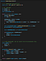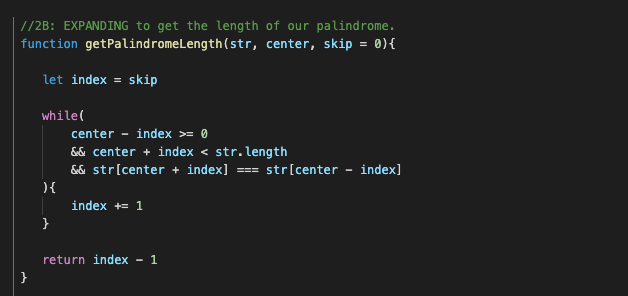# Finding the Longest Palindromic Substring With Manachar’s Algorithm in JavascriptYou after learning this Algo.

I have to admit. This algorithm is not easy to write about. However, I tried my best to distill the algorithm into understandable chunks, so that we can understand how the algorithm works.

We will use Manachar’s Algorithm to find the Longest Palindrome substring in O(n) complexity.

The following video is coded in Java, but it is a great explanation of what is actually happening in the algorithm. I suggest taking a look at the first half of this video.

Warning: This will not come easy. This is a bit complex. BUT if you stick with it we can get to a level of understanding that will hopefully help you code the solution.

There is a picture of the entire algorithm coded out at the bottom of this article! Please reference it as we go along.

We’re going to break it down into a few steps:

1. Seperation (preprocessing)
2. A: Iterate and B: Expand (while checking what we need to skip); add each palindrome length to an array
3. Getting the longest palindrome from the palindrome array (post processing)

** I’ve noted these steps in the screenshot of the algorithm.

# Separation

First, we separate each of our characters with a dummy character. It could be a ‘#’ or ‘@’, it doesn’t matter much.

So:

`abaabc`

will become

`#a#b#a#a#b#c#c#`

In Javascript we can do this with one line of code:

`const str = `#\${s.split("").join('#')}#``

Take note that this is increasing the size of the string to 2n + 1. This will be important when understanding the skip section.

# Iteration & Mirroring

See section 2A in Screenshot

Now that we have injected the hashes into our original string. We can iterate through each character and expand from each, checking for a palindrome as we do so.

See section 2B in Screenshot

We can make a function that check’s if we have a palindrome so that we are separating our concerns. (See last image in this article)

All this does is expands from the index and checks if there is a palindrome. We will talk about the skip in a second but first let’s see what we do with what we return from this helper function.

# Store Each palindrome’s length

We need an array to store each palindrome’s length after we iterate over it.

`const palindromes = []`

As we pass over each of the characters, we will expand to check if there is a palindrome around it.

If the palindrome is “#a#” we will count this as 1, since if you expand 1 index on each side you will have a palindrome.

`String : #a#b#a#a#b#c#c#P array: 010301010101010`

The magic of Manacher’s Algorithm lies in the skip value. This is where we are saving our processing power.

Much like how using memoization will cut down the amount of functions run in a recursive algorithm, this skip value will allow us to decide whether or not to Expand from the character we are currently iterating over. Or if we want to start the expansion from further out.

For example:

`String:    " # b # c # b # c # b  #  b  #"indexes:     0 1 2 3 4 5 6 7 8 9 10 11 12`

Let’s say we we have just expanded from the ‘b’ in bold. We now know that there is a palindrome extending from the first hash (0) to the second to last hash (10).

Now when we move to the next character ‘c’ (after the next #) we already know how far this first palindrome extends, and so we can start at the bounds instead of iterating over the “# b #” again.

In the long run this is going to save us a ton of expansion!

To get our skip value we will have to do a calculation that at first might be a bit confusing. But remember that adding “#” to the string makes the string 2n + 1.

`let skip = 1endOfWindow = center + windowif(i < endOfWindow){skip = Math.min(palindromes[2 * center - i], (endOfWindow - i))}`

We first set our skip as 1. But then make a check to decide to decide the minimum skip. We check here to see what our skip value should actually be. We take our current center value from the palindrome (which is from our window) and check if this is smaller than the end of window minus the current index.

This is hard to explain and wrap your mind around. But know that we’re trying to find our starting point for our helper function. This is where time is saved.

We’re essentially keeping track of a window that we’ve already checked this way we can make sure we’re not doing double work.

# Post Processing — Finding our answer

See section 3 in Screenshot

Once we’ve iterated through the entire array and kept track of the length of palindrome’s in each index, we need to return our actual answer.

This may be the most straight forward part of the algorithm. We are just iterating through our palindromes array and finding the longest length, then calculating the start index in our string array. Then we hit the string array and grab a substring from it with the start index and length.

`// 3: POST PROCESSING - get our answer from the palindromes array.const longest = {   start: 0,   length: 1}palindromes.forEach((length, index) => {if(length > longest.length){   longest.start = ((index - length) / 2)   longest.length = length }})return s.substr(longest.start, longest.length)`

Here is the function:This is not the entire Algo. Check the helper function to get the Palindrome length below.Here is our helper function which uses the skip value to determine where to begin comparing characters.

Software Developer — Game Creator — Let’s work together! Reach out on LinkedIn or Twitter @thedrewprint